NCERT Solutions: Basic Geometrical Ideas- 2

# NCERT Solutions: Basic Geometrical Ideas- 2 Notes | Study Mathematics (Maths) Class 6 - Class 6

## Document Description: NCERT Solutions: Basic Geometrical Ideas- 2 for Class 6 2022 is part of Mathematics (Maths) Class 6 preparation. The notes and questions for NCERT Solutions: Basic Geometrical Ideas- 2 have been prepared according to the Class 6 exam syllabus. Information about NCERT Solutions: Basic Geometrical Ideas- 2 covers topics like and NCERT Solutions: Basic Geometrical Ideas- 2 Example, for Class 6 2022 Exam. Find important definitions, questions, notes, meanings, examples, exercises and tests below for NCERT Solutions: Basic Geometrical Ideas- 2.

Introduction of NCERT Solutions: Basic Geometrical Ideas- 2 in English is available as part of our Mathematics (Maths) Class 6 for Class 6 & NCERT Solutions: Basic Geometrical Ideas- 2 in Hindi for Mathematics (Maths) Class 6 course. Download more important topics related with notes, lectures and mock test series for Class 6 Exam by signing up for free. Class 6: NCERT Solutions: Basic Geometrical Ideas- 2 Notes | Study Mathematics (Maths) Class 6 - Class 6
 1 Crore+ students have signed up on EduRev. Have you?

Exercise 4.4
Ques 1: Draw a rough sketch of a triangle ABC. Mark a point P in its interior and a point Q in its exterior. Is the point A in its exterior or in its interior?
Ans: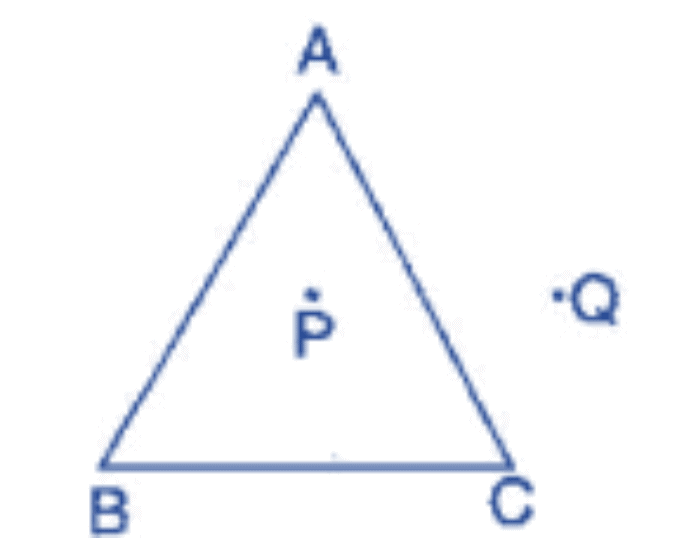A is neither interior of the figure nor exterior of triangle. It is a vertex
Ques 2: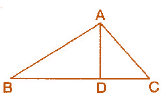(a) Identify three triangles in the figure.
(b) Write the names of seven angles.
(c) Write the names of six line segments.
(d) Which two triangles have ∠B as common?
Ans: (a) The three triangles are: ΔABC, ΔABD, ΔADC
(c) Line segments are: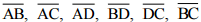(d) Triangles having common ∠B: ΔABC, ΔABD,

Exercise 4.5
Ques 1: Draw a rough sketch of a quadrilateral PQRS. Draw its diagonals. Name them. Is the meeting point of the diagonals in the interior or exterior of the quadrilateral?
Ans: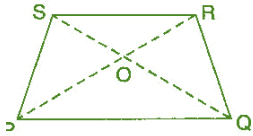Diagonal PR and diagonal SQ meet at O, which is inside the quadrilateral.

Ques 2: Draw a rough sketch of a quadrilateral KLMN. State,
(a) Two pairs of opposite sides.
(b) Two pairs of opposite angles.
(c) Two pairs of adjacent sides.
(d) Two pairs of adjacent angles.
Ans: (a) Pair of opposite sides: KL and MN, KN and LM
(b) Pair of opposite angles: ∠K and ∠M, ∠L and ∠N
(c) Pair of adjacent sides: KN and NM, KL and LM
(d) Pair of adjacent angles: ∠K and ∠N, ∠L and ∠M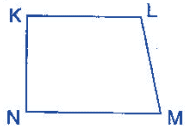Ques 3: Investigate:
Use strip and fasteners to make a triangle and a quadrilateral.
Try to push inward at any one vertex of the triangle. Do the same to the quadrilateral. Is the triangle distorted? Is the quadrilateral distorted? Is the triangle rigid?
Why is it that structures like electric towers make use of triangular shapes and not quadrilateral?
Ans: O is common to both the angles ∠AOC and ∠BOC.
No, the triangle is not distorted but the quadrilateral is distorted and also the triangle is rigid.
Structures like electric towers make use of triangular shape so that they could not be distorted and they could be rigid.

Exercise 4.6
Ques 1: From the figure, identify: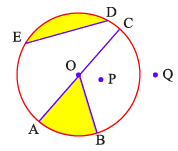(a) The centre of circle
(c) a diameter
(d) a chord
(e) Two points in the interior
(f) a point in the exterior
(g) a sector
(h) a segment
Ans: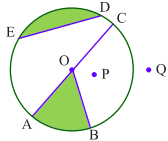(a) O is the centre.
(b) Three radii: OA, OB and OC
(c) A diameter: AC
(d) A chord: ED
(e) Interior points: O, P
(f) Exterior point: Q
(g) A sector: OAB
(h) A segment: ED

Ques 2: (a) Is every diameter of a circle also a chord?
(b) Is every chord of circle also a diameter?
Ans: (a) Yes, every diameter of a circle is also a chord.
(b) No, every chord of a circle is not a diameter.

Ques 3: Draw any circle and mark:
(a) Its centre.
(c) A diameter.
(d) A sector.
Ans: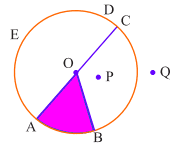(a) Its centre is O.
(b) A radius is OB.
(c) A diameter is AC.
(d) A sector OAB.

Ques 4: Say true or false:
(a) Two diameters of a circle will necessarily intersect.
(b) The centre of a circle is always in its interior.
Ans: (a) True
(b) True

The document NCERT Solutions: Basic Geometrical Ideas- 2 Notes | Study Mathematics (Maths) Class 6 - Class 6 is a part of the Class 6 Course Mathematics (Maths) Class 6.
All you need of Class 6 at this link: Class 6

## Mathematics (Maths) Class 6

167 videos|275 docs|43 tests
 Use Code STAYHOME200 and get INR 200 additional OFF

## Mathematics (Maths) Class 6

167 videos|275 docs|43 tests

Track your progress, build streaks, highlight & save important lessons and more!

,

,

,

,

,

,

,

,

,

,

,

,

,

,

,

,

,

,

,

,

,

;